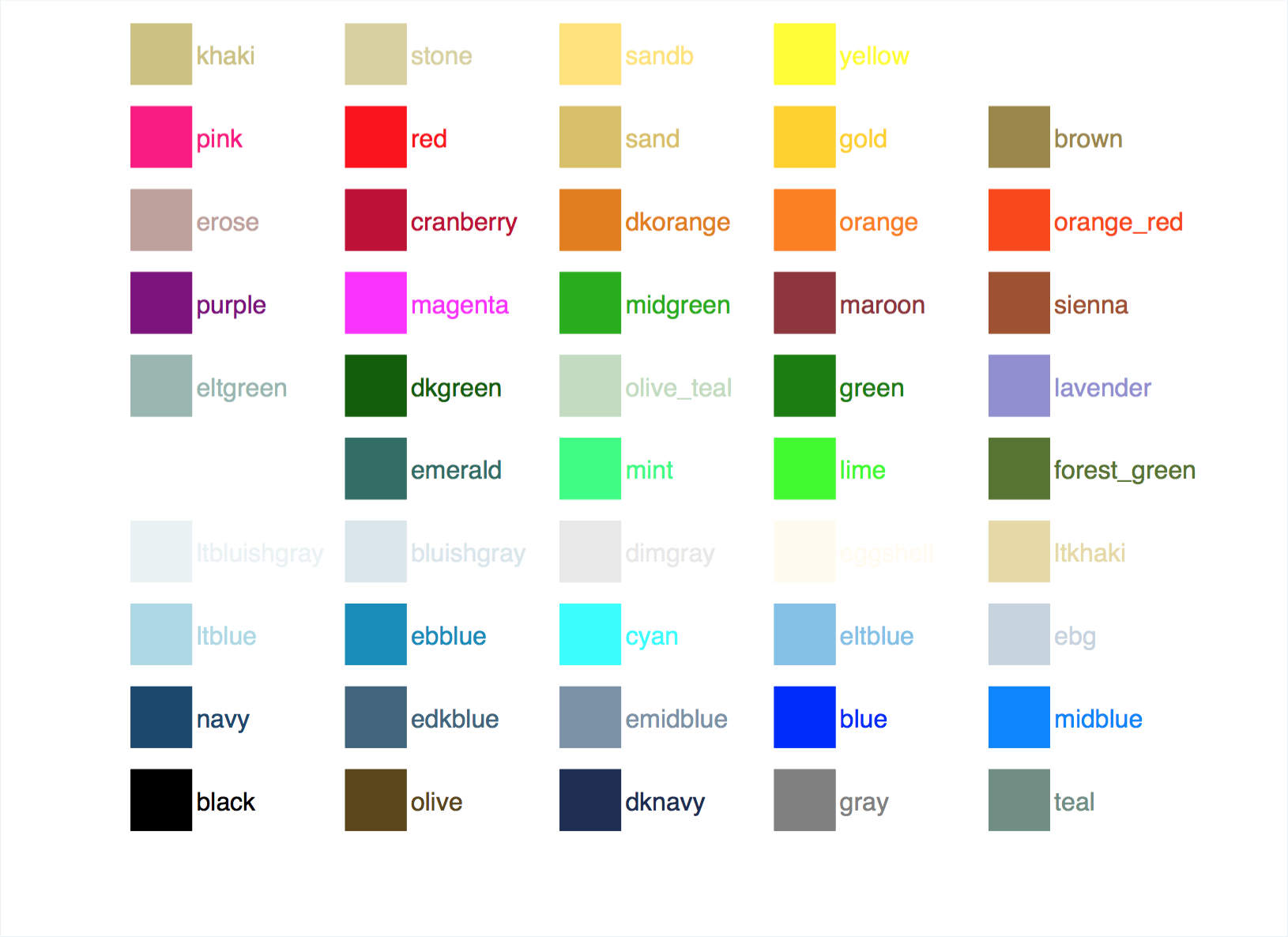# Stata Tips & Snippets

This is a live version of my personal Stata Tips & Snippets file. I wrote this for myself as a reference, and I thought that it might be helpful for others.

Table of contents:

## Dates and times

### Date types in Stata

#### Summary tables

Date type Examples of HRFs
datetime `20jan2010 09:15:22.120`
date `20jan2010, 20/01/2010, ...`
weekly date `2010w3`
monthly date `2010m1`
quarterly date `2010q1`
half-yearly date `2010h1`
yearly date `2010`

SIF type Functions to build from components
datetime/c `tc = mdyhms(M, D, Y, h, m, s)`
`tc = dhms(td, h, m, s)`
`tc = hms(h, m, s)`
datetime/C `tC = Cmdyhms(M, D, Y, h, m, s)`
`tC = Cdhms(td, h, m, s)`
`tC = Chms(h, m, s)`
date `td = mdy(M, D, Y)`
weekly date `tw = yw(Y, W)`
monthly date `tm = ym(Y, M)`
quarterly date `tq = yq(Y, Q)`
half-yearly date `th = yh(Y, H)`
yearly date `ty = y(Y)`

From ↓ To datetime/c To datetime/C To date
datetime/c `tC = Cofc(tc)` `td = dofc(tc)`
datetime/C `tc = cofC(tC)` `td = dofC(tC)`
date `tc = cofd(td)` `tC = Cofd(td)`
weekly `td = dofw(tw)`
monthly `td = dofm(tm)`
quarterly `td = dofq(tq)`
half-yearly `td = dofh(th)`
yearly `td = dofy(ty)`

Source: Stata documentation

HRF = human readable form

SIF = Stata internal form

### Converting strings to SIF date

``````// String format: 2016-09-28T09:53:38
gen double newvarname = clock(varname, "20YMD#hms")
format newvarname %tc

// String format: 2016-09-28 09:53:38
gen double newvarname = clock(varname, "20YMDhms")
format newvarname %tc

// String format: 2016-09-28
gen double newvarname = date(varname, "20YMD")
format newvarname %td``````

### Extracting hours, minutes, and seconds from SIF dates

``````gen hours = hh(tcvar)
gen minutes = mm(tcvar)
gen seconds = ss(tcvar)
gen dayofweek = dow(dofc(tcvar))
gen month = month(dofc(tcvar))
gen year = year(dofc(tcvar))``````

### Getting the last day of a given month

``````local yearmonth = ym(2016,2)

di %td dofm(`yearmonth' + 1) - 1
// 29feb2016

di day(dofm(`yearmonth' + 1) - 1)
// 29
``````

## Working with missing data

Use `missing(varname1, varname2)` rather than `== .` or `== ""`. Details here.

## Reading MySQL data directly into Stata

``````clear
set odbcdriver ansi
odbc list
odbc load, table("tablename") dsn("mysql") clear noquote``````

Or you can do:

``odbc load, exec("select * from tablename") dsn("mysql") clear noquote``

Other resources:

## Using `bysort` to run commands over levels of a categorical variable

For example, if you have a categorical variable `gender` and a continuous variable `height`, and you want to run `summarize` by `gender` (`summarize` doesn’t support `by`), you can do this:

``bysort gender: summarize height``

## Add group counts to dataset

If you want to fill in the `n_group` column in a dataset like this…

id group n_group
1 1 3
2 1 3
3 1 3
4 2 2
5 2 2

…you can use this command: `egen n_group = count(group), by(group)`

## Add column total variable

This will sum a column and generate a new variable (the same for each row) with the total:

``egen sumvar = sum(var)``

## Counting the number of distinct values of a variable

``````* Option 1
tab varname, nofreq
display r(r)

* Option 2
inspect varlist
display r(N_unique)

* Option 3
codebook varlist``````

(Source: statdaily.com)

## Create value labels from another variable

``````net install labutil
labmask integervarname, values(stringvarname)``````

## Flow control

### For loops

``````foreach i in 0 1 2 {
di `i'
}
* 0
* 1
* 2

foreach i in a b c {
di "`a'"
}
* a
* b
* c

forval i = 0/2 {
di `i'
}
* 0
* 1
* 2``````

If you want to loop through all levels of a variable:

``````levelsof varname, local(levels)
foreach l of local levels {
whatever if varname == `l'
}``````

## Auto-complete variable names in Sublime Text

I like writing Stata code in Sublime Text, but I miss variable auto-completion. To get a poor man’s version of this, run the `ds` command in Stata, and then copy and paste the results into a `/* */` comment block in your `.do` file in Sublime.

## ColorsCourtesy of the palette_all command.

## Splitting up long lines

There are two ways to do this:

``````* Option 1
regress lnwage educ complete age c.age#c.age     ///
exp c.exp#c.exp tenure c.tenure#c.tenure ///
i.region female

* Option 2 (only works in do-files and ado-files)
#delimit ;
regress lnwage educ complete age c.age#c.age
exp c.exp#c.exp tenure c.tenure#c.tenure
i.region female ;
predict e, resid ;
#delimit cr``````

See `help delimit` for more details.

## Reloading `.ado` programs

Use the `-discard-` command and then run `-program_name-`. (source)

## Working with very large datasets

Stata suggests looking at `ftools` and `gtools`.

## Suggestions? Corrections?

Please contact me.